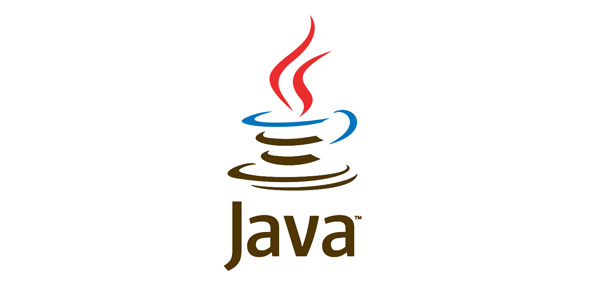# Itemp Java Quiz 3

10 Questions | Attempts: 180
ShareSettingsChapter 3 Revision

• 1.
Which of the following is NOT a reserved word in Java?
• A.

Import

• B.

Finally

• C.

Friend

• D.

Goto

• 2.
Which of the following integer primitive types can correctly represent a value of 65000?
• A.

Short

• B.

Int

• C.

Byte

• D.

All of these

• 3.
Which of the following statements declaring and initializing boolean primitive variables would not be rejected by the Java compiler?
• A.

Boolean flag = true;

• B.

Boolean flag = true;

• C.

Boolean flag = TRUE;

• D.

Boolean flag = TRUE;

• 4.
Which of the following variable names will be rejected by the Java compiler?
• A.

Animal

• B.

2ndletter

• C.

_me2you

• D.

\$price

• 5.
Which of the following is the range of values that a byte can store?
• A.

-127 to 127

• B.

-127 to 128

• C.

-128 to 127

• D.

0 to 255

• 6.
Which of the following uses the same number of bits as the int primitive variable?
• A.

Byte

• B.

Float

• C.

Long

• D.

Double

• 7.
How many bits does a Java double primitive value use?
• A.

32 bits

• B.

48 bits

• C.

64 bits

• D.

128 bits

• 8.
1. lightspeed = 1816000;
2. days = 1000;
3. seconds = days * 24 * 60 * 60;
4. distance = lightspeed * seconds;
When this block of code is executed, what will be the value of variable seconds after the execution of line 3 ?
• A.

1816000

• B.

1000

• C.

86400000

• D.

156200000000

• 9.
What will be the value assigned to variable answer after executing this code?
• A.

0

• B.

Null

• C.

50

• D.

51

• 10.
1. byte b = 50;
2. b = b * 2; What will happen if you try to compile this block of code?
• A.

It will compile successfully

• B.

It will give error: Cannot assign an int to a byte!

• C.

It will give error because the answer is above the limit of a byte

• D.

It will give error because a byte is not suitable for multiplication.

## Related Topics

×

Wait!
Here's an interesting quiz for you.